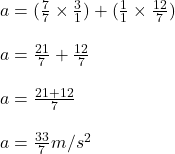## A student solving for the acceleration of an object has applied appropriate physics principles and obtained the expression a = a1 + Fm where

Question

A student solving for the acceleration of an object has applied appropriate physics principles and obtained the expression a = a1 + Fm where a1 = 3.00 m/s2, F = 12.0 kg.m/s2 and m = 7.00 kg. First, which of the following is the correct step for obtaining a common denominator for the two fractions in the expression in solving for a?
a. (m/m times a1/1) + (1/1 times F/m).
b. (1/m times a1/1) + (1/m times F/m).
c. (m/m times a1/1) + (F/F times F/m).
d. (m/m times a1/1) +(m/m times F/m ).

in progress 0
3 months 2021-07-19T16:32:47+00:00 1 Answers 132 views 0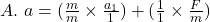Explanation:

Given the expression for calculating the acceleration as shown;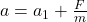where;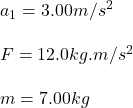To get the correct step for obtaining a common denominator for the two fractions in the expression in solving for a, we will find the LCM of  the given expression as shown;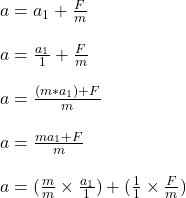The final expression gives the requires step. On substituting the given parameters into the resulting expression to get a;